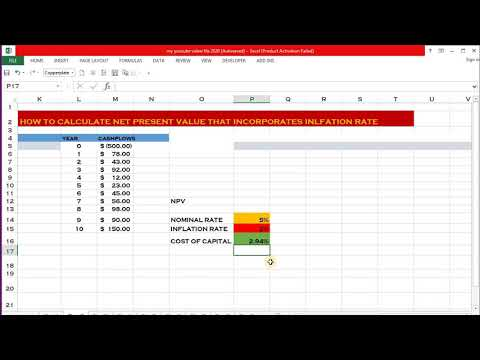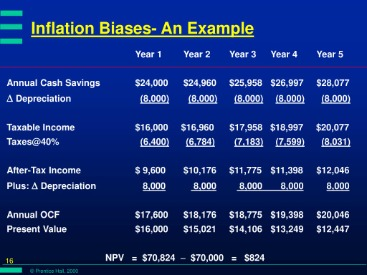# Problem-6: Net present value NPV analysis with inflationNet present value (NPV) represents the sum of all future cash flows over the entire life of a project or investment that is discounted to the present day. Company ABC is considering an investment proposal which requires making an initial investment of \$ 40 million. The project expects to generate future cash of \$10 million per year for 5 years. The nominal discounted rate is 5% and the inflation rate is 2% per year. The difference from the first method, this method uses normal future cash flow and normal discounted rate to calculate the NPV. The expected future cash flow must be adjusted with inflation before discount.

In the nominal method, nominal project cash flows are discounted at nominal discount rate. Using variable rates over time, or discounting “guaranteed” cash flows differently from “at risk” cash flows, may be a superior methodology but is seldom used in practice. Using the discount rate to adjust for risk is often difficult to do in practice (especially internationally) and is difficult to do well.NPV uses discounted cash flows to account for the time value of money. As long as interest rates are positive, a dollar today is worth more than a dollar tomorrow because a dollar today can earn an extra day’s worth of interest. Even if future returns can be projected with certainty, they must be discounted for the fact that time must pass before they’re realized—time during which a comparable sum could earn interest. Time value of money dictates that time affects the value of cash flows. This decrease in the current value of future cash flows is based on a chosen rate of return (or discount rate).

## What Is The Net Present Value (NPV Calculator) of a Lump Sum Payment Discounted for Inflation?

If, for example, the capital required for Project A can earn 5% elsewhere, use this discount rate in the NPV calculation to allow a direct comparison to be made between Project A and the alternative. Re-investment rate can be defined as the rate of return for the firm’s investments on average. When analyzing projects in a capital constrained environment, it may be appropriate to use the reinvestment rate rather than the firm’s weighted average cost of capital as the discount factor.

No matter how the discount rate is determined, a negative NPV shows that the expected rate of return will fall short of it, meaning that the project will not create value. For consistency, an investment should be appraised using the same methods across different calculations. If you use a nominal interest rate, you should first discount future cash flows for the inflation effect. A firm’s weighted average cost of capital (after tax) is often used, but many people believe that it is appropriate to use higher discount rates to adjust for risk, opportunity cost, or other factors. A variable discount rate with higher rates applied to cash flows occurring further along the time span might be used to reflect the yield curve premium for long-term debt.

## Example of Calculating NPV

We can use the same equation to determine the inflation rate, real interest rate, or nominal interest rate when the other two components are known. Another advantage of the net present value method is its ability to compare investments. As long as the NPV of each investment alternative is calculated back to the same point in time, the investor can accurately compare the relative value in today’s terms of each investment.

• It compares the present value of money today to the present value of money in the future, taking inflation and returns into account.
• Inflation is the increase of prices that generally leads to a decline in the value of money.
• NPV is determined by calculating the costs (negative cash flows) and benefits (positive cash flows) for each period of an investment.
• This way, you’ll receive consistent appraisal value of future cash flows.
• The NPV formula assumes that the benefits and costs occur at the end of each period, resulting in a more conservative NPV.
• For example, present value is used extensively when planning for an early retirement because you’ll need to calculate future income and expenses.

It accounts for the fact that, as long as interest rates are positive, a dollar today is worth more than a dollar in the future. Meanwhile, today’s dollar can be invested in a safe asset like government bonds; investments riskier than Treasurys must offer a higher rate of return. However it’s determined, the discount rate is simply the baseline rate of return that a project must exceed to be worthwhile. The net present value (NPV) or net present worth (NPW) applies to a series of cash flows occurring at different times. The present value of a cash flow depends on the interval of time between now and the cash flow.

## NPV and Inflation

If for example there exists a time series of identical cash flows, the cash flow in the present is the most valuable, with each future cash flow becoming less valuable than the previous cash flow. A cash flow today is more valuable than an identical cash flow in the future because a present flow can be invested immediately and begin earning returns, while a future flow cannot. Another approach to choosing the discount rate factor is to decide the rate which the capital needed for the project could return if invested in an alternative venture.

• In such cases, that rate of return should be selected as the discount rate for the NPV calculation.
• NPV is used in capital budgeting and investment planning to analyze the profitability of a projected investment or project.
• As long as interest rates are positive, a dollar today is worth more than a dollar tomorrow because a dollar today can earn an extra day’s worth of interest.

In general, projects with a positive NPV are worth undertaking while those with a negative NPV are not. M2 SWF is considering a project that is expected to generate \$10 million at the end of each year for 5 years. Where r is the discount rate and c1, c2, up to Cn represent cash flows for respective years and Xo is the initial investment. If the present value of these cash flows had been negative because the discount rate was larger or the net cash flows were smaller, then the investment would not have made sense.

It means when future cash flows are discounted with a higher discount rate, the net present value of the future cash flows decreases. The full calculation of the present value is equal to the present value of all 60 future cash flows, minus the \$1 million investment. The calculation could be more complicated if the equipment was expected to have any value left at the end of its life, but in this example, it is assumed to be worthless. The internal rate of return (IRR) is calculated by solving the NPV formula for the discount rate required to make NPV equal zero. This method can be used to compare projects of different time spans on the basis of their projected return rates. We can use the NPV of this project by discounting the future cash flows to their present value terms and by adjusting them for inflation.

## Net Present Value (NPV) with Inflation

Since the future can never be known there is always an element of uncertainty to the calculation despite the the scientific accuracy of the calculation itself. Present value can also be used to give you a rough idea of the amount of money needed at the start of retirement to fund your spending needs. You’ll then compare that to what you have saved now – or what you think you’ll have saved by your retirement date – and that gives you a rough idea of whether your savings is on track or not.

It compares the present value of money today to the present value of money in the future, taking inflation and returns into account. Because of its simplicity, NPV is a useful tool to determine whether a project or investment will result in a net profit or a loss. A positive NPV results in profit, while a negative NPV results in a loss.

This Present Value Calculator makes the math easy by converting any future lump sum into today’s dollars so that you have a realistic idea of the value received. Both methods will give the same result, if not there must something wrong with the calculation. As both calculations have included inflation with just different methods. It means a higher inflation rate means a lower interest rate and vice versa. The net present value method can be used in several ways to determine the financial feasibility of a project, viability of an investment, business valuation, and so on.

However, in practical terms a company’s capital constraints limit investments to projects with the highest NPV whose cost cash flows, or initial cash investment, do not exceed the company’s capital. NPV is a central tool in discounted cash flow (DCF) analysis and is a standard method for using the time value of money to appraise long-term projects. It is widely used throughout economics, financial analysis, and financial accounting. After all, the NPV calculation already takes into account factors such as the investor’s cost of capital, opportunity cost, and risk tolerance through the discount rate. And the future cash flows of the project, together with the time value of money, are also captured. Therefore, even an NPV of \$1 should theoretically qualify as “good,” indicating that the project is worthwhile.